# Appendix A

## A1.0 Introduction

The primary purpose of this appendix is to derive the differential equations presented in Section 3.2. Implicit in this derivation are several underlying assumptions:

• The fate and transport of the modeled solute can be described by a one-dimensional system.
• Model parameters may be spatially and temporally variable. Spatial variation is assumed to occur in the longitudinal direction only.
• The mechanisms shown in Table 1 influence solute behavior in the stream channel and the storage zone, as indicated.
• The volume of the storage zones does not change in time.

Mechanistic models such as the one presented in this report are based on a fundamental engineering principle, conservation of mass. This principle states that for a finite volume of water, mass is neither created or destroyed (see Chapra and Reckhow, 1983). In short, mass conservation implies that: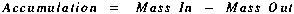(A1)

Equation (A1) is often called a mass balance equation. This mass balance must be applied to a finite volume of water, known as a control volume. A control volume for our one-dimensional system is shown below. Note that the terms 'segment' (introduced in Section 3.2) and 'control volume' can be used interchangeably. In figure A1,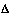x represents the segment length, while `1' and `2' are used to demarcate the upstream and downstream boundaries of the control-volume.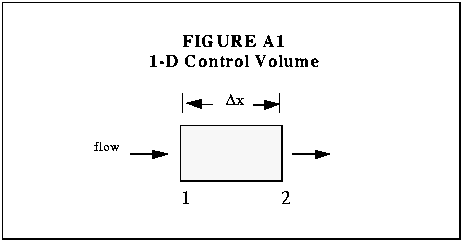The remainder of this appendix is devoted to the formulation of differential equations based on Equation (A1). To accomplish this task, we first define `Accumulation' and then proceed to develop the `in' and `out' terms for each process given in Table 1. This procedure is carried out for both the stream channel and the storage zone.

## A2.0 Mass Balance - Stream Channel

### I. Accumulation

By definition, accumulation is the change in mass with respect to time. For the main stream channel, accumulation is given by: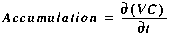(A2)

where

 C in-stream solute concentration [M/L3] V volume of the main channel segment [L3] t time [T]
We can now expand Equation (A2) using the chain rule, yielding: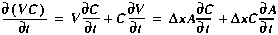(A3)

wherex segment length [L] A stream cross-sectional area [L2]

We now begin to develop the `in' and `out' terms for each process. The mass advected into the control volume, i.e. across the upstream boundary, is given by: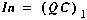(A4)

where Q is the volumetric flowrate [L3/T].

Next, the mass advected out of the control volume, i.e. across the downstream boundary, is given by: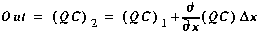(A5)

Finally, we combine the `In' and `Out' terms, giving: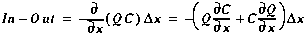(A6)

### III. Dispersion

In a similar fashion, we define the dispersive flux across the upstream and downstream boundaries: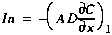(A7)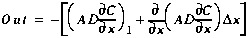(A8)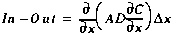(A9)

where D is the dispersion coefficient [L2/T].

### IV. Lateral Flows

Here we are interested in mass entering the system via lateral inflow, and mass leaving through lateral outflow. Note that unlike the previous two mechanisms, the concentrations associated with mass inflows and outflows differ (CL vs. C):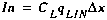(A10)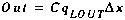(A11)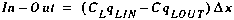(A12)

where

 CL solute concentration of the lateral inflow [M/L3] qLIN lateral inflow rate [L3/T-L] qLOUT lateral outflow rate [L3/T-L]

### V. Transient Storage

The final physical mechanism we consider for the stream channel is transient storage. The inflow and outflow terms are given by: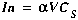(A13)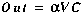(A14)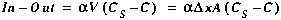(A15)

wherestorage zone exchange coefficient [/T] CS storage zone solute concentration [M/L3]
Note that the exchange coefficient,, is defined as the fraction of the main channel volume that is exchanged with the storage zone, per unit time.

### VI. Accumulation = In - Out

Now that the individual processes have been elucidated, the results from I-V may be used to develop the mass balance (Equation (A1)). Setting the accumulation term equal to the combined `In - Out' terms and dividing byx gives: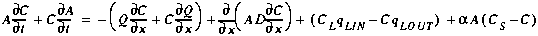(A16)

Although Equation (A16) represents a mass balance for a one-dimensional stream, it may be simplified using the water balance shown below.

### VII. Water Balance

Like the solute mass balance presented above, a water mass balance is based on conservation of mass. Here again we develop Equation (A1) for a one-dimensional control volume or stream segment. Using the assumption of incompressible flow, accumulation is given by: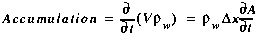(A17)

where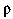w is the density of water [M/L3].

The inflow term for the water balance is the sum of the water advected across the upstream interface and the water entering via lateral inflow: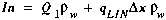(A18)

Similarly, outflow is the sum of the water advected out and that leaving through lateral outflow: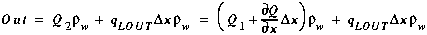(A19)

Finally, the water balance is completed by combining Equation (A17) through (A19), and dividing bywx: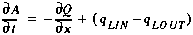(A20)

### VIII. Governing Equation - Stream Channel

Substituting Equation (A20) into Equation (A16) and dividing by A yields the governing differential equation for the stream channel: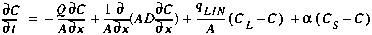(A21)

This equation is presented in Section 3.2.1 as Equation (1).

## A3.0 MASS BALANCE - STORAGE ZONE

As in Section A2.0, a mass balance procedure is used to develop the governing equation. Here the approach is greatly simplified, as the processes of advection, dispersion and lateral inflow do not affect the storage zone.

### I. Accumulation

Accumulation of solute mass in the storage zone is given by:(A22)

where

 VS volume of the storage zone segment [L3] AS storage zone cross-sectional area [L2]
Note that the storage zone area, AS, is assumed to be constant in time.

### II. Transient Storage

The change in storage zone solute mass is given by: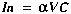(A23)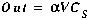(A24)(A25)

whereis the fraction of the main channel volume that is exchanged with the storage zone, per unit time.

### III. Governing Equation - Storage Zone

Combining Equations (A22) and (A25) we arrive at: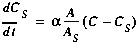(A26)

This governing equation is presented in Section 3.2.1 as Equation (2).